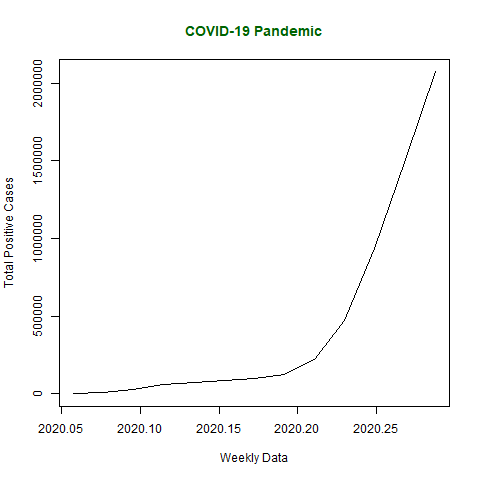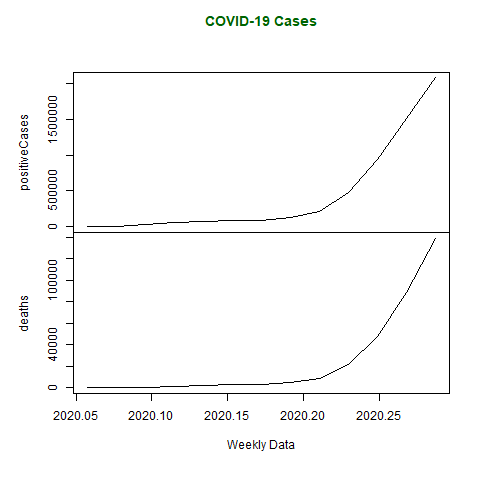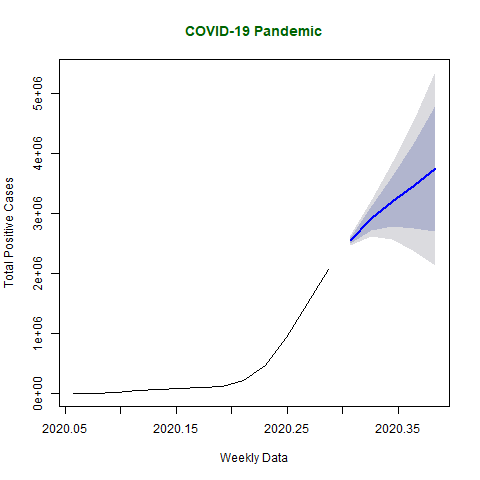Open in App
Not now

## Related Articles

• Write an Interview Experience

# Time Series Analysis in R

• Difficulty Level : Easy
• Last Updated : 28 Feb, 2023

Time Series Analysis in R is used to see how an object behaves over a period of time. In R Programming Language, it can be easily done by the ts() function with some parameters. Time series takes the data vector and each data is connected with a timestamp value as given by the user. This function is mostly used to learn and forecast the behavior of an asset in business for a period of time. For example, sales analysis of a company, inventory analysis, price analysis of a particular stock or market, population analysis, etc.

Syntax:  objectName <- ts(data, start, end, frequency)

where,

• data – represents the data vector
• start – represents the first observation in time series
• end – represents the last observation in time series
• frequency – represents number of observations per unit time. For example, frequency=1 for monthly data.

Note: To know about more optional parameters, use the following command in the R console:

`help("ts")`

## Examples of Time Series Analysis

Let’s take the example of the COVID-19 pandemic situation. Taking the total number of positive cases of COVID-19 cases weekly from 22 January 2020 to 15 April 2020 the world in data vector.

## R

 `# Weekly data of COVID-19 positive cases from``# 22 January, 2020 to 15 April, 2020``x <- ``c``(580, 7813, 28266, 59287, 75700,``    ``87820, 95314, 126214, 218843, 471497,``    ``936851, 1508725, 2072113)` `# library required for decimal_date() function``library``(lubridate)` `# output to be created as png file``png``(file =``"timeSeries.png"``)` `# creating time series object``# from date 22 January, 2020``mts <- ``ts``(x, start = ``decimal_date``(``ymd``(``"2020-01-22"``)),``                            ``frequency = 365.25 / 7)` `# plotting the graph``plot``(mts, xlab =``"Weekly Data"``,``        ``ylab =``"Total Positive Cases"``,``        ``main =``"COVID-19 Pandemic"``,``        ``col.main =``"darkgreen"``)` `# saving the file``dev.off``()`

Output:Time Series Data visualization chart

## Multivariate Time Series Analysis

Multivariate Time Series is creating multiple time series in a single chart. Taking data of total positive cases and total deaths from COVID-19 weekly from 22 January 2020 to 15 April 2020 in a data vector.

## R

 `# Weekly data of COVID-19 positive cases and``# weekly deaths from 22 January, 2020 to``# 15 April, 2020` `positiveCases <- ``c``(580, 7813, 28266, 59287,``                ``75700, 87820, 95314, 126214,``                ``218843, 471497, 936851,``                ``1508725, 2072113)` `deaths <- ``c``(17, 270, 565, 1261, 2126, 2800,``            ``3285, 4628, 8951, 21283, 47210,``            ``88480, 138475)` `# library required for decimal_date() function``library``(lubridate)` `# output to be created as png file``png``(file=``"multivariateTimeSeries.png"``)` `# creating multivariate time series object``# from date 22 January, 2020``mts <- ``ts``(``cbind``(positiveCases, deaths),``start = ``decimal_date``(``ymd``(``"2020-01-22"``)),``                    ``frequency = 365.25 / 7)` `# plotting the graph``plot``(mts, xlab =``"Weekly Data"``,``    ``main =``"COVID-19 Cases"``,``    ``col.main =``"darkgreen"``)` `# saving the file``dev.off``()`

Output:Multivariate Time Series Analysis using R

## Time Series Forecasting

Forecasting can be done on time series using some models present in R. In this example, Arima automated model is used. To know about more parameters of arima() function, use the below command.

`help("arima")`

In the below code, forecasting is done using the forecast library and so, installation of the forecast library is necessary.

## R

 `# Weekly data of COVID-19 cases from``# 22 January, 2020 to 15 April, 2020``x <- ``c``(580, 7813, 28266, 59287, 75700,``    ``87820, 95314, 126214, 218843,``    ``471497, 936851, 1508725, 2072113)` `# library required for decimal_date() function``library``(lubridate)` `# library required for forecasting``library``(forecast)` `# output to be created as png file``png``(file =``"forecastTimeSeries.png"``)` `# creating time series object``# from date 22 January, 2020``mts <- ``ts``(x, start = ``decimal_date``(``ymd``(``"2020-01-22"``)),``                            ``frequency = 365.25 / 7)` `# forecasting model using arima model``fit <- ``auto.arima``(mts)` `# Next 5 forecasted values``forecast``(fit, 5)` `# plotting the graph with next``# 5 weekly forecasted values``plot``(``forecast``(fit, 5), xlab =``"Weekly Data"``,``ylab =``"Total Positive Cases"``,``main =``"COVID-19 Pandemic"``, col.main =``"darkgreen"``)` `# saving the file``dev.off``()`

Output :

```         Point Forecast   Lo 80   Hi 80   Lo 95   Hi 95
2020.307        2547989 2491957 2604020 2462296 2633682
2020.326        2915130 2721277 3108983 2618657 3211603
2020.345        3202354 2783402 3621307 2561622 3843087
2020.364        3462692 2748533 4176851 2370480 4554904
2020.383        3745054 2692884 4797225 2135898 5354210```

Below graph plots estimated forecasted values of COVID-19 if it continues to be widespread for the next 5 weeks.Time Series Forecasting using R

My Personal Notes arrow_drop_up